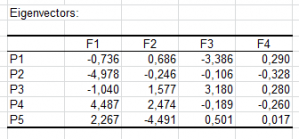# Principal Coordinate Analysis

Principal Coordinate Analysis (PCoA) is used to visualize proximity matrices. Available in Excel using the XLSTAT add-on statistical software.## Principal Coordinate Analysis

Principal Coordinate Analysis (PCoA) is a powerful and popular multivariate analysis method that lets you analyze a proximity matrix, whether it is a dissimilarity matrix, e.g. a euclidean distance matrix, or a similarity matrix, e.g. a correlation matrix.

XLSTAT provides a PCoA feature with several standard options that will let you represent your data efficiently and gain a deep insight on them:

• Run a PCoA on a similarity or a dissimilarity matrix
• Correct negative eigenvalues if needed using the Square root or Lingoes correction
• Filter factors by fixing a maximum number of axes to be retained or by fixing a minimum of variance explained

## What is Principal Coordinate Analysis?

Principal Coordinate Analysis (often referred to as PCoA) is aimed at graphically representing a resemblance matrix (similarity matrix or dissimilarity matrix) between p elements (individuals, variables, objects, among others).

The algorithm can be divided into three steps:

1. Computation of a distance matrix, e.g. a euclidean distance matrix, for the p elements
2. Centering of the matrix by rows and columns
3. Eigen-decomposition of the centered distance matrix

The rescaled eigenvectors correspond to the principal coordinates (principal axes), which are synthetic variables, that can be used to display the p objects in a space with 1, 2, ... , p-1 dimensions.

As with PCA (Principal Component Analysis) eigenvalues can be interpreted in terms of percentage of total variability that is being represented in a reduced space.

## Results of Principal Coordinate Analysis in XLSTAT

• Delta1 matrix: This matrix corresponds to the D1 matrix of Gower, used to compute the eigen-decomposition.
• Eigenvalues and percentage of inertia: this table displays the eigenvalues and the corresponding percentage of inertia.
• Principal coordinates: This table displays the principal coordinates of the objects that are used to create the chart where the proximities between the charts can be interpreted.
• Contributions: This table displays the contributions that help evaluate how much an object contributes to a given axis.
• Squared cosines: This table displays the contributions that help evaluate how close an object is to a given axis.

### Principal Coordinate Analysis and Principal Component Analysis

PCA and Principal Coordinate Analysis are quite similar in the way that the PCA can also represent observations in a space with less dimensions, the latter being optimal in terms of carried variability. A Principal Coordinate Analysis applied to matrix of Euclidean distances between observations (calculated after standardization of the columns using the unbiased standard deviation) leads to the same results as a PCA based on the correlation matrix. The eigenvalues obtained with the Principal Coordinate Analysis are equal to (p-1) times those obtained with the PCA.

## Principal Coordinate Analysis and Multidimensional Scaling

Principal Coordinate Analysis and MDS (Multidimensional Scaling) share the same goal of representing objects for which we have a proximity matrix.

MDS has two drawbacks when compared with Principal Coordinate Analysis:

• The algorithm is much more complex and performs slower.
• Axes obtained with MDS cannot be interpreted in terms of variability.

MDS has two advantages compared with Principal Coordinate Analysis:

• The algorithm allows having missing data in the proximity matrix.
• The non-metric version of MDS provides a simpler and clear way to handle matrices where only the ranking of the distances is important.

## Tutorial on how to run PCoA in Excel using the XLSTAT software

Here is an example on how to run a Principal coordinate analysis (PCoA) with XLSTAT.### analice sus datos con xlstat

prueba gratuita de 14 días

Incluido en